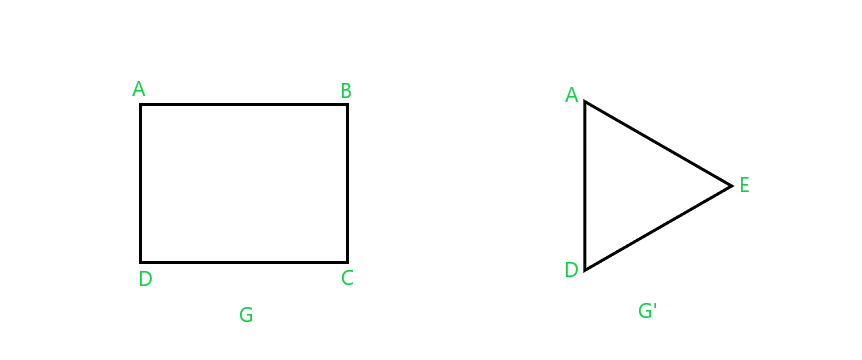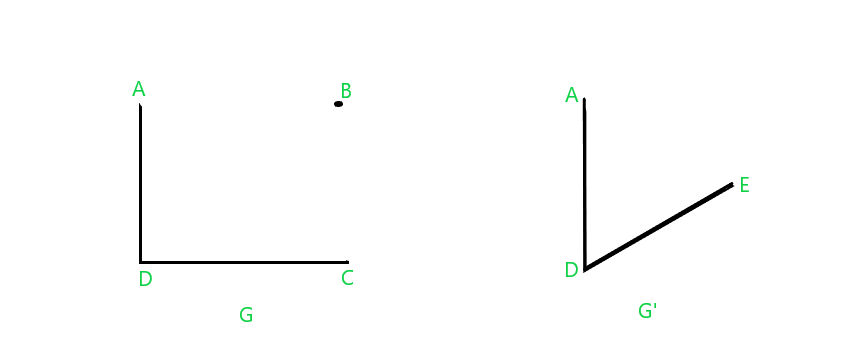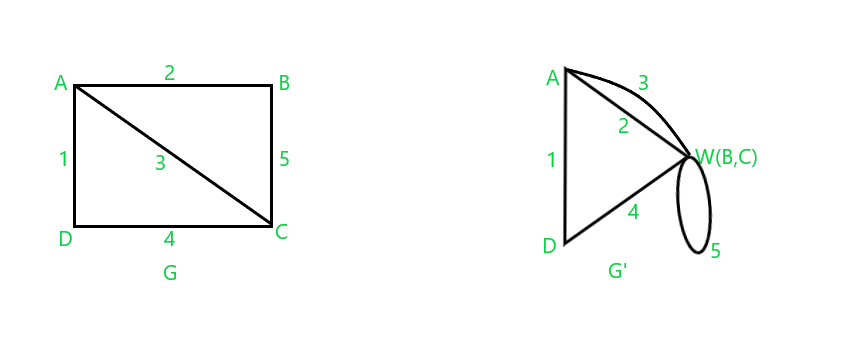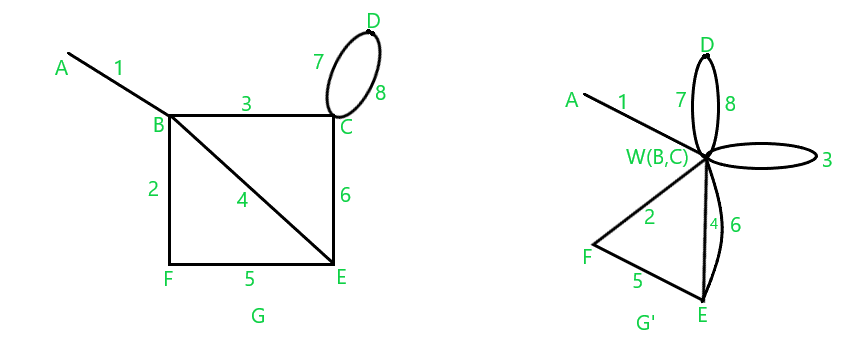Open in App
Not now

# Fusion Operation In Graph

• Last Updated : 24 Jan, 2023

### What is Fusion operation in Graph Theory?

Let u and v be two distinct vertices in graph G. The fusion of the vertices u and v are treated as a single new vertex ‘w’ and every edge which was incident on u and v, are now incident on ‘w‘.

So if a graph has n number of vertices, then the resultant fused graph will have n-1 number of vertices. Let us see some examples of the fuse operation in graph theory.

### Examples of Fusion in Graph:

Example 1: Simple GraphFusion changing number of both edges and vertices

In the above example of fusion operation, the vertices B and C of graph G are fused into a vertex E in graph G’. Also, note that the number of vertices and edges was reduced from four to three. But although the number of vertices will always be reduced by one in fusion operation, we cannot say the same about edges.

Example 2: Vertices reduced but edges remain sameFusion without changing number of edges

In the above example, we had 3 edges in graph G and 3 edges in graph G’ too after the fusion of vertices B and C into E.

Example 3: Fusion creating self-loop

The task is to apply the fusion operation to the vertices B and C of graph G to fuse them into the vertex W in the resultant graph G’ maintaining all the edges as in the original graph G.Fusion creating a self-loop

As we can see in the above fusion operation, vertices B and C have been fused into vertex W with all the edges that were incident on vertices B and C, now incident upon vertex W.

Example 4: Fusion in graph with self-loop

In this example also, the task is to apply the fusion operation to the vertices B and C of graph G to fuse them into the vertex W in the resultant graph G’.Fusion in Graph with self-loop

As we can see in the above fusion operation, vertices B and C have been fused into vertex W with all the edges that were incident on vertices B and C, now incident upon vertex W.

My Personal Notes arrow_drop_up
Related Articles